# PostgreSQL索引分析记录之B树及其变种树B+、B*介绍

2014/10/09 20:52

## 一、B树定义

1、B树（定义1引自《算法导论（第三版）》）：

一棵B树T是具有一下性质的有根树(根为T.root)：
（1）每个结点x具有下面属性：
a.x.n，当前存储在结点x中的关键字个数。
b.x.n个关键字本身，x.key1x.key2x.key3x.key4，……，x.keyn，以非降序存放，使得x.key1<=x.key2<=……<=x.keyn
c.x.leaf，一个布尔值，如果x是叶子结点，则为true；如果x是内部结点，则为false
（2）每个内部结点x还包含x.n+1个指向其孩子的指针x.c1x.c2，……，x.cn。叶子结点没有孩子，所以它们的ci属性没有定义。

（3）关键字x.keyi对存储在各子树中的关键字加以分割：如果k为任意一个存储在以x.ci为根的自述中的关键字，那么k为任意一个存储在以x.ci为根的子树中的关键字，则：
k1<=x.key1<=k2<=x.key2<=……<=kn<=x.keyn<=kn+1
（4）每个叶子结点具有相同的深度，即树的高度h
（5）每一个结点能包含的关键字数由一个上界和下界。这些界可用一个称作B树的最小度数t>=2来表示。
a.每个费根的结点必须至少有t-1个关键字。每个非根的内结点至少有t个子女。如果树是非空的，则根结点至少包含一个关键字。
b.每个结点可包含至多2t-1个关键字。所以一个内结点至多可有2t个子女。我们说一个结点是满的，如果它恰好有2t-1个关键字。

2、B树（定义2引自《计算机程序设计艺术（第三卷）（第三版）》）：

阶m的一株B树，是满足一下性质的一株树：
（1）每个结点至多有m个儿子。
（2）除了根和叶之外，每个结点的儿子个数至少是m/2（取上整）。
（3）根至少有两个儿子（除非它是一片叶（叶子结点））。
（4）所有叶都出现在同一级，而且不带信息。
（5）具有k个儿子的非叶子结点含有k-1个键码（关键字）。

3、B树（定义3引自百度百科）：

一棵m阶B树(balanced tree of order m)是一棵平衡的m路搜索树。它或者是空树，或者是满足下列性质的树：
（1）根结点至少有两个子女；
（2）每个非根结点所包含的关键字个数 j 满足：┌m/2┐ - 1 <= j <= m - 1；
（3）除根结点以外的所有结点（不包括叶子结点）的度数正好是关键字总数加1，故内部子树个数 k 满足：┌m/2┐ <= k <= m ；
（4）所有的叶子结点都位于同一层。
在B-树中，每个结点中关键字从小到大排列，并且当该结点的孩子是非叶子结点时，该k-1个关键字正好是k个孩子包含的关键字的值域的分划。
因为叶子结点不包含关键字，所以可以把叶子结点看成在树里实际上并不存在外部结点，指向这些外部结点的指针为空，叶子结点的数目正好等于树中所包含的关键字总个数加1。
B-树中的一个包含n个关键字，n+1个指针的结点的一般形式为： （n,P0,K1,P1,K2,P2,…,Kn,Pn）

其中，Ki为关键字，K1<K2<…<Kn, Pi 是指向包括Ki到Ki+1之间的关键字的子树的指针。

4、B树（定义4《数据结构（C语言版）》（2007年版））：

一棵阶的 B-树，或为空树，或为满足下列特性的叉树：
（1）树中每一个结点至多有棵子树；
（2）若根结点不是叶子结点，则至少有两棵子树；
（3）除根结点之外的所有非终端结点至少有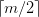棵子树；
（4）所有的非终端结点中包含下列信息数据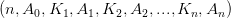， 其中：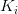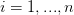）为关键字，且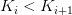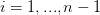）；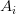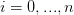）                              指向子树根结点的指针，且指针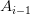所指子树中所有结点的关键字均小于），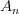所指子树中所有结点的关键字均大于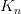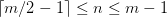）为关键字的个数（或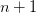为子树个数）；
（5）所有的叶子结点都出现在同一层次上，并且不带信息（可以看作是外部结点或查找失败的结点，实际上这些结点不存在，指向这些结点的指针为空）。

B树的结构：

#define t 2
typedf struct BTNode{
int	n;
KeyType	key[2*t];
struct BTNode *c[2*t + 1];
bool leaf;
}BTNode, *BTree;

B树的模型：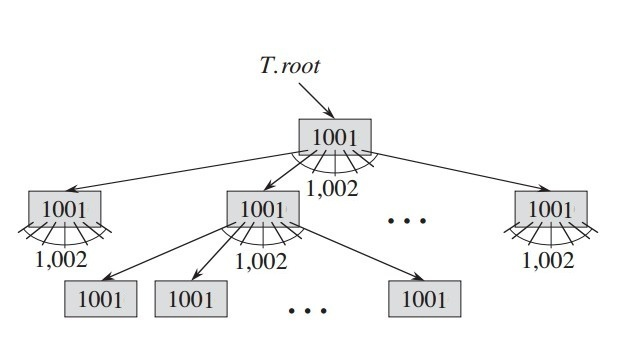## 二、B树的操作

### 1、创建一棵空的B树

/*
* B树的新建函数，通过对B树 T的根结点进行操作
*/

B-TREE-CREATE(T)
{
/* ALLOCATE-NODE()为新结点x分配磁盘页 */
x = ALLOCATE-NODE();
/* 默认结点为叶子结点 */
x.leaf = TRUE;
x.n = 0;
DISK-WRITE(x);
/* 当前树的根结点为新建结点x */
T.root = x;
}

### 2、搜索B树

/*
* 寻找k值得结点 * 以x为根结点，进行搜寻
*/
B-TREE-SEARCH(x, k)
{
i = 1;
/* 线性搜索，比较当前结点所有的关键字 */
while i <= x.n and k > x.key[i]
i++;
/* 确认是否找到关键字，找到则返回当前结点以及坐标 */
if i <= x.n and k == x.key[i]
return(x, i);
/* 否则判断是否为叶子结点，如果是，则返回null，表示无法找到 */
else if x.leaf
return NIL;
/* 若既不是叶子结点，也不在当前结点内，则根据性质搜寻子结点 */
else
return B-TREE_SEARCH(x.c[i], k);
}

### 3、向B树插入关键字

#### 1）分裂函数

/*
* x为满结点的父结点，i是满结点在父结点中的位置。
* 当结点为满结点时，即子结点为2t，关键字为2t - 1，
* 分裂时，以第t各关键字为中心，两边分别变为有t - 1个关键字，t个子结点的结点 * 将第t个关键字提到父结点中（由于遇到满结点就分裂，buxu）
*/
B-TREE-SPLIT-CHILD(x, i)
{
/* 建立新的结点z，z作为分裂结点中的左结点 */
z = ALLOCATE-NODE();
/* zh */
y = x.c[i];
/* 为新结点z初始化 */
z.leaf = y.leaf;
z.n = t - 1;
/* 将满结点中的后t-1个关键字赋值到新结点z中 */
for j = 1 to t - 1
z.key[i] = y.key[j + t];
/* 如果y不是叶子结点那么，将满结点y中的后t个子结点赋值到新建结点z中 */
if not y.leaf
for j = 1 to t;
z.c[j] = y.c[j + t];
/* 修改y中的关键字个数 */
y.n = t - 1;
/* 将父结点x中的i之后（不包括i）位置的子结点向后移动一位 */
for j = x.n + 1 downto i + 1
x.c[j + 1] = x.c[j];
/* 将父结点x中的i+1位置的子结点赋值为z */
x.c[i + 1] = z;
/* x中的关键字自i后（包括i）关键字位置向后移动一位 */
for j = x.n downto i
x.key[j + 1] = x.key[i];
/* 将子结点的关键字赋值到父结点中 */ x.key[i] = y.key[t];
/* 关键字个数发生改变 */
x.n = x.n + 1;
/* 对修改进行写磁盘 */
DISK-WRITE(y);
DISK-WRITE(z);
DISK-WRITE(x);
}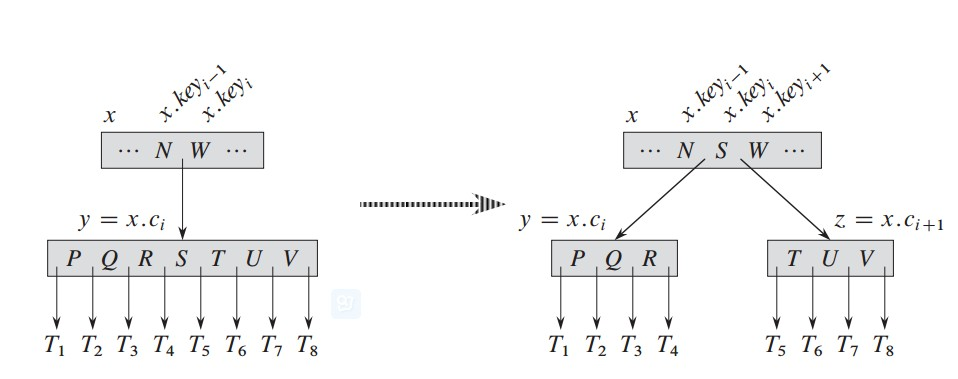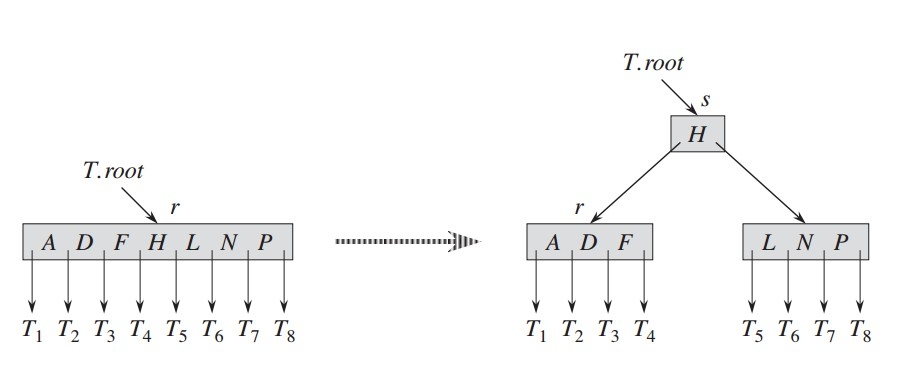#### 2）对非满结点进行插入

/*
* 假定x是非满结点 * 当是叶子结点且非满时，直接寻找位置进行插入
* 否则寻找子结点（首先判断是否为满结点）进行插入
*/
B-TREE-INSERT-NONFULL(x, k)
{
/*
* 将x结点的关键字个数赋值给i，这是为了后边的查找位置
*（个人认为这里的循环查找可以变为二分查找）
*/
i = x.n;
/* 当当前结点为叶子结点且非满时，寻找位置插入 */
if x.leaf
while i >= 1 and k < x.key[i]
x.key[i + 1] = x.key[i];
i--;
x.key[i + 1] = k;
x.n = x.n + 1;
DISK-WRITE(x);
/*
* 当当前结点不为叶子结点且非满时，寻找对应的子结点 * 先对子结点进行判断，当为满时，进行分裂，否则递归插入
*/
else while i >= 1 and k < x.key[i]
i--;
i++;
if x.c[i].n == 2*t - 1;
B-TREE-SPLIT-CHILD(x, i);
if k > x.key[i];
i++;
B-TREE-INSERT-NONFULL(x.c[i], k);
}

#### 3）插入函数

/*
* 插入函数，所有插入操作都经过此函数
* T为整棵树，k为关键字
*/
B-TREE-INSERT(T, k)
{
/* 先将树T的根结点赋值给r */
r = T.root;
/* 判断树T的根结点是否为满结点，是的话新建父结点 * 进行分裂操作，然后进行插入操作
*/
if r.n == 2*t - 1
s = ALLOCATE-NODE();
T.root = s;
s.leaf = FALSE;
s.n = 0;
s.c = r;
B-TREE-SPLIT-CHILD(s, 1);
B-TREE-INSERT-NONFULL(s, k);
/* 否则直接进行非满结点插入 */
else B-TREE-INSERT-NONFULL(r, k);
}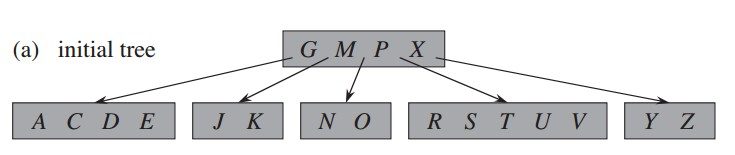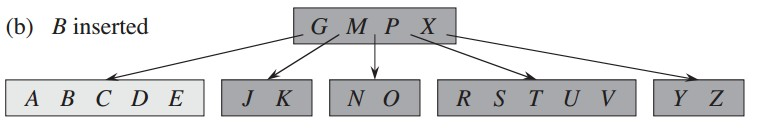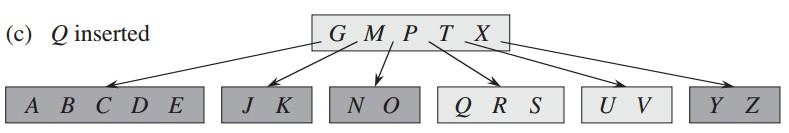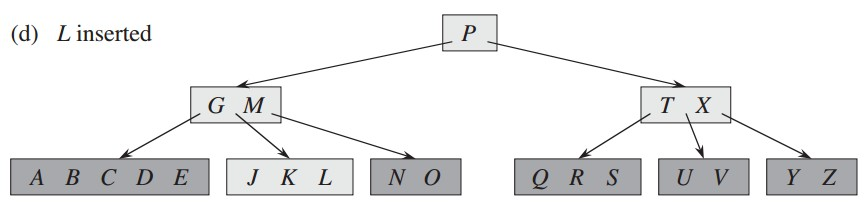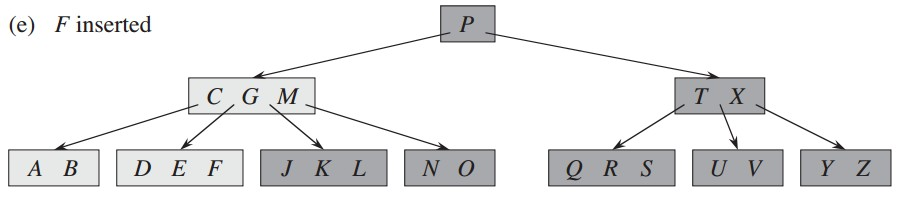### 4、删除关键字

#### 1）语言描述

1）如果关键字k在结点x中，而且x是个叶结点，则从x中删除k。
2）如果关键字k在结点x中，而且x是个内结点，则作如下操作：
a.如果结点x中前于k的子结点y包含至少t个关键字，则找出k在以y为根的子树种的前驱k'。递归地删除k'，并在x中用k'取代k。
b.对称的,如果y有少于t个关键字,则检查结点x中后于k的子结点z.如果z至少有t个关键字,则找出k在以z为根的子树中的后继k'.递归的删除k',并在x中用k'代替k.
c.否则，如果y和z都只含有t-1个关键字，则将k和z的全部合并进y，这样x就失去了k和指向z的指针，并且y现在包含2*t-1个关键字。然后释放z并递归地从y中删除k。
3）如果关键字k当前不在内部结点x中，则确定必包含k的子树的根x.c[i]（如果k确实在树种）。如果x.c[i]只有t-1个关键字，必须执行步骤3a或3b来保证降至一个至少包含t个关键字的结点。然后，通过对x的某个合适的子结点进行递归而结束。
a.如果x.c[i]只含有t-1个关键字，但是它的一个相邻的兄弟至少包含t个关键字，则将x中的某一个关键字降至x.c[i]中，将x.c[i]的相邻的左兄弟或右兄弟的一个关键字升至x，将该兄弟中相应的孩子指针移到x.c[i]中，这样就使得x.c[i]增加一个额外的关键字。
b.如果x.c[i]以及x.c[i]的所有相邻兄弟都只包含t-1个关键字，则将x.c[i]与一个兄弟合并，即将x的一个关键字移至新合并的结点，使之成为该结点的中间关键字。

#### 2）过程代码

/*
* 将后继结点或者右兄弟的某个关键字、父结点的某个关键字以及该结点某个关键字进行移动。
* 移动后，注意被移动结点的子结点的移动。
* x父结点，y当前结点，z被移动的结点，i关键字在x关键字中的位置
* 该函数是情形为被移动的结点在该结点的左边
*/
B-TREE-LEFT(x, y, z, i)
{
y.key[y.n+1] = x.key[i];
x.key[i] = z.key;
for j = 1 to z.n - 1
z.key[j] = z.hey[j+1];
if not z.leaf
y.c[y.n+2] = z.c;
for j = 1 to n
z.c[j] = z.c[j+1];
y.n =y.n+1;
z.n = z.n-1;
free(z.c[j + 1]);
free(z.key[j]);
}
/*
* 将后继结点或者右兄弟的某个关键字、父结点的某个关键字以及该结点某个关键字进行移动。
* 移动后，注意被移动结点的子结点的移动。
* x父结点，y当前结点，z被移动的结点，i关键字在x关键字中的位置
* 该函数是情形为被移动的结点在该结点的右边
*/
B-TREE-RIGHT(x, y, z, i)
{
for j = n to 1
y.key[j+1] = y.hey[j];
y.key = x.key[i];
x.key[i] = z.key[z.n];
if not y.leaf
for j = n+1 to 1
y.c[j+1] = y.c[j];
y.c = z.c[z.n+1];
z.n = z.n - 1;
y.n = y.n + 1;
free(z.c[z.n+1]);
free(z.key[z.n]);
}

/*
* 合并两个子结点 */
B-TREE-MERGE-CHILD(x, i)
{
if i == x.n
i--;
y = x.c[i];
z = x.c[i+1];
y.key[t] = x.key[i];
for j = 1 to z.n
y.key[t+j] = z.key[j];
if not z.leaf
for j = 2 to z.n + 2
y.c[t+j] = z.[j-1];
for j = i to x.n-1
x.key[i] = x.key[i+1];
x.n = x.n - 1;
if not x.n

return i;
}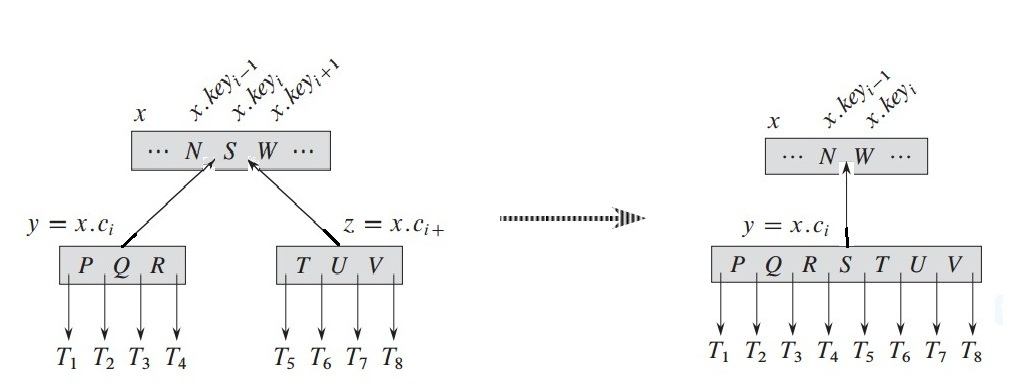/*
* 删除叶子结点的关键字
*/
B-TREE-DELETE-LEAF(x, i)
{
for j = i to x.n - 1
x.key[j] = x.key[j+1];
free(x.key[j+1]);
}
/*
* 删除内结点关键字
*/
B-TREE-DELETE-IN(x, i)
{
y = x.c[i];
z = x.c[i+1];

if y.n >= t
k' = TREE-MAXIMUM(y);
key' = x.key[i];
x.key[i] = k'.key[k'.n];
k'.key[k'.n] = key';
else if z.n >= t
k' = TREE-MINIMUM(z);
key' = x.key[i];
x.key[i] = k'.key;
k'.key = key';

i++;
else  i = B-TREE-MERGE-CHILD(x,i);

return i;
}

/*
* 删除树T的关键字key
*/
B-TREE-DELETE(T, key)
{
x= T.root;
/* 寻找关键字位置，或者下降的子结点位置 */
while i < x.n and key > x.key[i]
i++;

/* 若再该层且为叶子结点删除结点，否则下降寻找结点删除 */
if i < x.n and key == x.key[i]
if(x.leaf)
B-TREE-DELETE-LEAF(x, i);
else
{
i = B-TREE-DELETE-IN(x, i);

B-TREE-DELETE(x.c[i], key);
}
else
{
if x.leaf
printf("there is no the key %d!!\n", key);
else
{
if x.c[i].n >= t
{
B-TREE-DELETE(x.c[i], key);
}
else
{
if i > 0 and x.c[i - 1].n >= t
{
B-TREE-RIGHT(x, x.c[i], x.c[i - 1], i);
}
else if i < x.n and x.c[i + 1].n >= t
B-TREE-LEFT(x, x.c[i], x.c[i + 1], i);
else
i = B-TREE-MERGE-CHILD(x, i);

B-TREE-DELETE(x.c[i], key);
}
}
}
}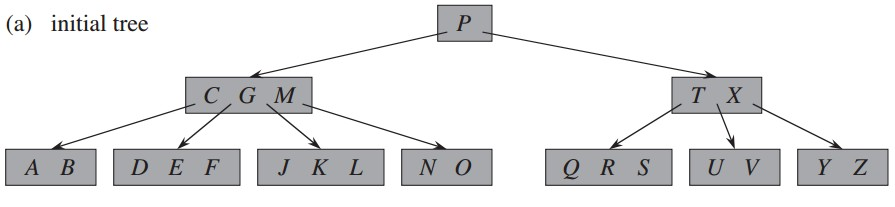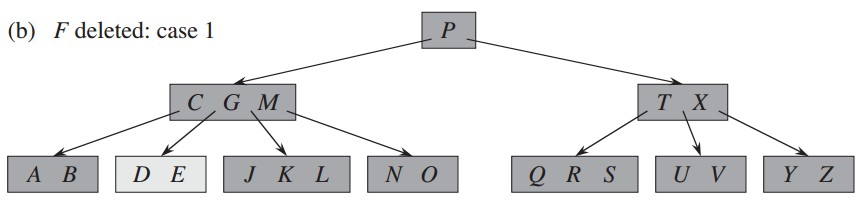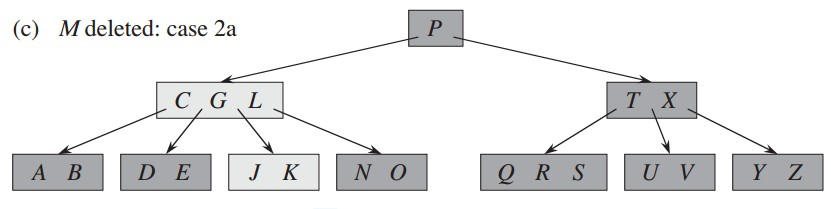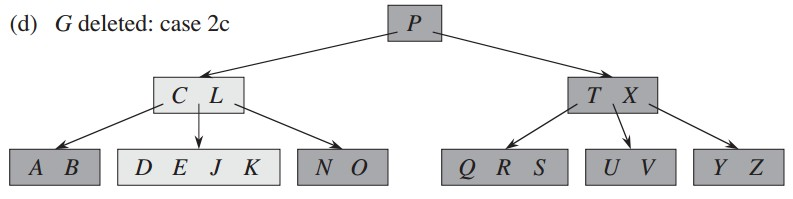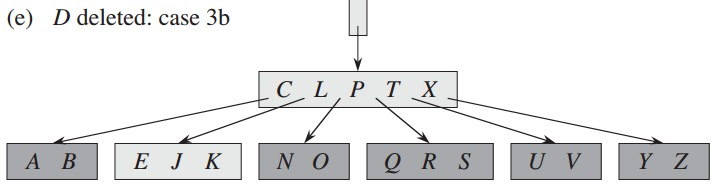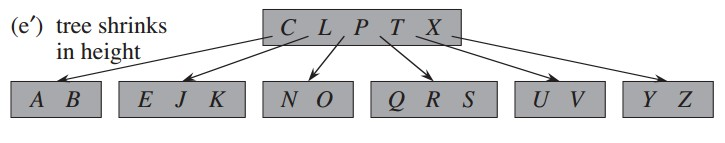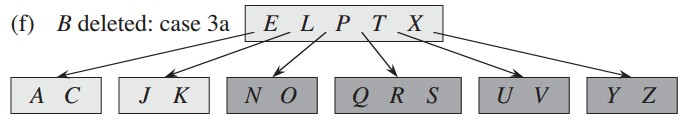/*
* 如果是叶子结点，则返回第一个关键字，否则递归调用
*/
TREE-MINIMUM(x)
{
if islevel
return (x, 1);
if x.leaf
return (x, 1);
return TREE-MINIMUM(x.c);
}

/*
* 如果是叶子结点，则返回最后一个关键字，否则递归调用
*/
TREE-MAXIMUM(x)
{
if islevel
return (x, n);
if x.leaf
return x;
return TREE-MAXIMUM(x.c[x.n+1]); }

## 三、定理证明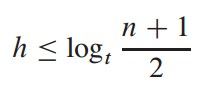由B树性质可以得知，根结点最少有两个子结点，其他结点除叶子结点都至少有t个子结点，所以有深度为1时有当前层为2个，深度为2时，当前层为2t个结点，深度为3时为2t^2,以此类推，当为深度为h，当前层有2t^(h-1),所以：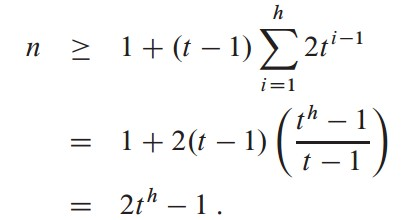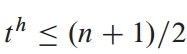## 四、支持第一种定义的原因

1、插入：首先进行插入关键字操作，当关键字个数为m时，该结点为缺陷点，对缺陷点进行分裂，否则插入完成。这就有一个问题，即需要回溯到父结点进行分裂，而当父结点同样为缺陷点后，需要进行再次回溯，这样最坏的情况就需要回溯到根结点。

2、删除：类似于前边的插入操作，先删除，发现该结点为缺陷点（即n小于m/2-1）,进行调整，然后回溯，这样就同样会出现，回溯到根结点的情况。

## 五、变种树介绍

### 1、B+树

#### B+树的定义

B+树整体和B树结构类似，是B树的变体，结点结构不一样，从而导致分裂操作不同。
1、其内部结点结构和B树相同。
2、叶子结点最后一个指针为兄弟结点的指针，其他指针指向真正数据。
3、其次B+树中的内部结点都是关键字索引，所有数据都存储在叶子结点内。
4、B+树有两个头指针，分别是根结点的指针以及叶子结点最小关键字的指针。

#### B+树相对于B树的优点

1) B+-tree的磁盘读写代价更低
B+-tree的内部结点并没有指向关键字具体信息的指针。因此其内部结点相对B 树更小。如果把所有同一内部结点的关键字存放在同一盘块中，那么盘块所能容纳的关键字数量也越多。一次性读入内存中的需要查找的关键字也就越多。相对来说IO读写次数也就降低了。
2) B+-tree的查询效率更加稳定

3)数据库索引采用B+树的主要原因是 B树在提高了磁盘IO性能的同时并没有解决元素遍历的效率低下的问题。正是为了解决这个问题，B+树应运而生。B+树只要遍历叶子结点就可以实现整棵树的遍历。而且在数据库中基于范围的查询是非常频繁的，而B树不支持这样的操作（或者说效率太低）。

### 2、B*树

#### B*树的定义

B*树是B+树的变体，在B+树的基础上，在内部结点增加指向兄弟结点的指针，其次，关键字个数至少是最大值的2/3。

1、空间使用率提高。
2、减少了分裂操作。

0
0 收藏

### 作者的其它热门文章0 评论
0 收藏
0